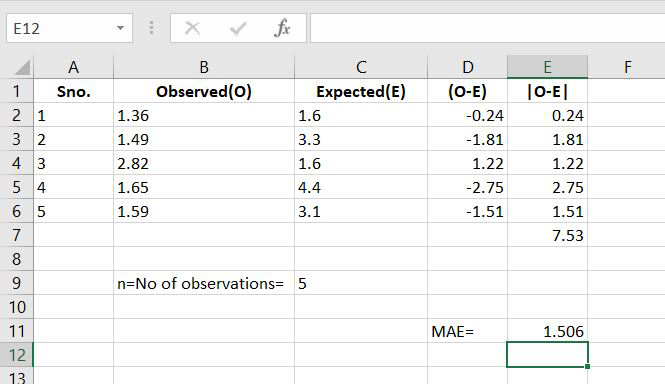Open In App

# How to Calculate Mean Absolute Error in Excel?

Mean absolute error is the measure of error between the observed and the expected values in a given data set.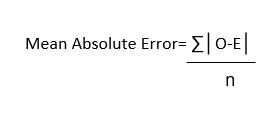Where,

• O stands for Observed values,
• E stands for Expected values,
• n stands for total no. of observations.

Now let us understand it with the help of an example.

Example:

Follow the below steps to calculate MAE in Excel:

Step 1: Suppose we have the following data: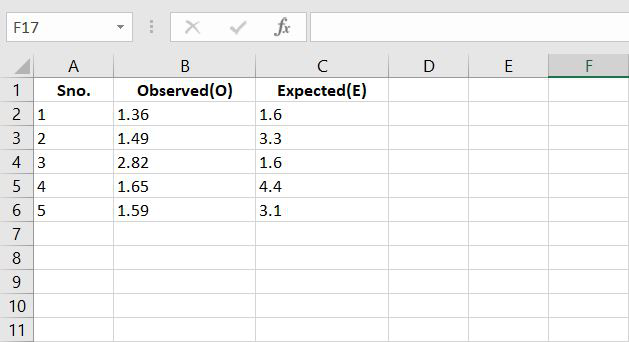Step 2: According to formulae, let’s calculate the difference between the observed and the expected values.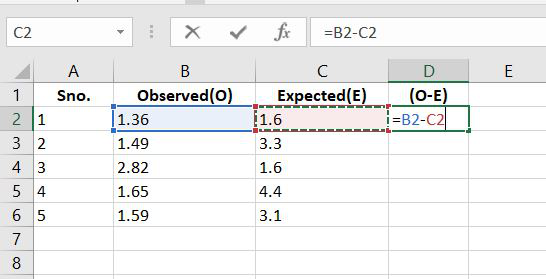Step 3: Now let’s calculate the absolute values.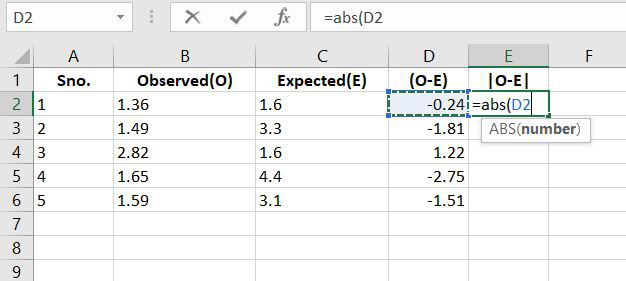Step 4: Now we know from the data that n= no of observations and in this the value of n=5. And also the let’s calculate the total sum of |O-E|.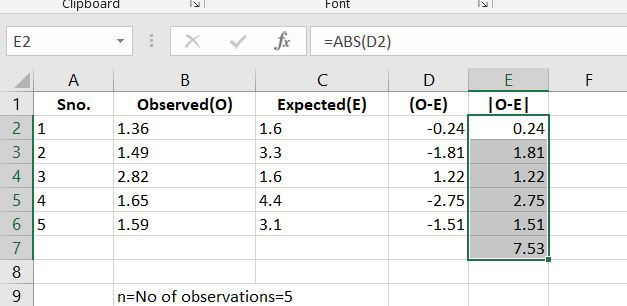Step 5: Now we have the value of Sum of |O-E| and the value of n. Now according to formulae, the Mean Absolute Error is calculated as: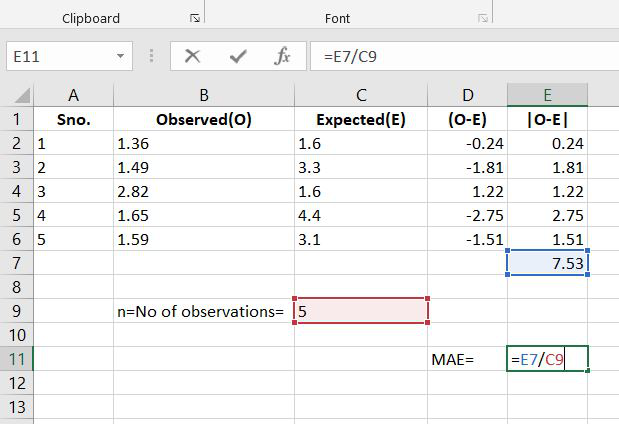Step 6: So the value of MAE is: